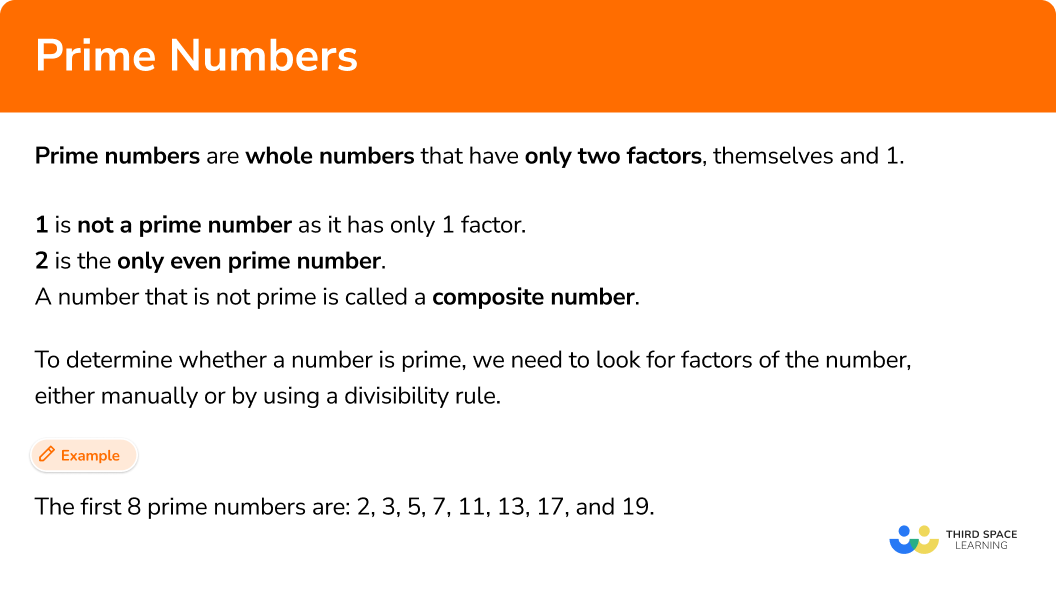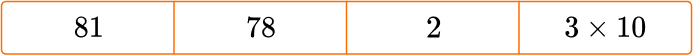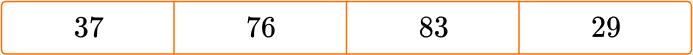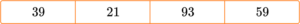# Prime numbers

Here we will learn about prime numbers, including what they are, how we can determine whether a number is prime, and how to solve problems that involve prime numbers.

Students will first learn about prime numbers as part of operations and algebraic thinking in elementary school.

## What are prime numbers?

Prime numbers are whole numbers that have only two factors, themselves and 1.

This means that you cannot divide a prime number by any number apart from 1 or itself, and get a whole number as an answer.

A number that is not prime is called a composite number.

1 is not a prime number as it has only 1 factor.

2 is the only even prime number.

To determine whether the number is prime, check whether it has any factors other than itself and 1, either manually or by using a divisibility rule. If the number has a factor that is not itself or 1, it is not prime.

There are a few useful divisibility rules that can help us determine whether a number is divisible by a whole number and therefore has that whole number as a factor.

FactorDivisibility rulesExample
2 The last digit is a 2, 4, 6, 8, or 0
(an even number).
1154 is divisible by 2 as the last digit is a 4.
3 The sum of the digits is a multiple of 3. 357 is divisible by 3 as 3+5+7=15
and 15 is a multiple of 3.
5 The last digit is 5 or a 0. 64854260 is divisible by 5 as the last digit is a 0.
6 The sum of the digits is a multiple of 6. 11109 is divisible by 6 as 1+1+1+0+9=12
which is a multiple of 6.
9 The sum of the digits is a multiple of 9. 5931 is divisible by 9 as 5+9+3+1=18
which is a multiple of 9.
10 The last digit is a 0. 640 is divisible by 10 as the last digit is a 0.

### What are prime numbers?## Common Core State Standards

How does this relate to 4th grade math and 5th grade math?

• Grade 4: Operations and Algebraic Thinking (4.OA.B.4)
Find all factor pairs for a whole number in the range 1–100. Recognize that a whole number is a multiple of each of its factors. Determine whether a given whole number in the range 1– 100 is a multiple of a given one-digit number. Determine whether a given whole number in the range 1–100 is prime or composite.

## How to find prime numbers

In order to determine whether a number is a prime number:

1. Use the divisibility rules to see whether 2, 3 or 5 is a factor.
2. If they are not factors, test for divisibility by 7 and 8.
3. State your conclusion with a reason.

## Prime numbers examples

### Example 1: two digit number

Is 53 a prime number?

1. Use the divisibility rules to see whether 2, 3 or 5 is a factor.

The last digit is not a 2, 4, 6, 8, or 0 so it is not a multiple of 2.

Adding the digits together we have 5+3=8 and so it is not a multiple of 3.

The last digit is not a 5 or a 0 and so it is not a multiple of 5.

2If they are not factors, test for divisibility by 7 and 8.

We need to divide 53 by 7 and 8, using long division, until we reach the first number that gives a quotient that is a whole number.

Start by dividing by 7\text{:}

As 53 \div 7=7 \; r4 \; 7 is not a factor of 53.

Next divide by 8\text{:}

As 53 \div 8=6 \; r5 \; 8 is not a factor of 53

The number 53 is not divisible by 7 or 8, so there are no more whole numbers that we need to try.

3State your conclusion with a reason.

53 is a prime number as it only has two factors, 1 and 53.

### Example 2: determine the prime number from a list of numbers

One of the following numbers is prime. Identify the prime number.

The last digit of 14 is a 4 and so 14 is a multiple of 2.

The last digit of 25 is a 5 and so 25 is a multiple of 5.

9 is a multiple of 3.

The final two numbers are 61 and 84. As 61 is a smaller number, it is easiest to determine whether 61 is prime.

61 does not end in a 2, 4, 6, 8, or 0 and so 61 is not a multiple of 2.

6+1=7 and so 61 is not a multiple of 3.

61 does not end in a 5 or 0 and so it is not a multiple of 5.

We need to see whether 61 is divisible by either 7 or 8.

61\div 7=8 \; r 5

61 \div 8=7 \; r 5

61 is not divisible by 7 or 8 and is therefore prime.

61 is the prime number as it only has 2 factors, 1 and 61.

Note: 84=7 \times 12

### Teaching tips for prime numbers

• Fluency with multiplication facts can assist students when trying to determine if a number is a composite or prime number.

• Instead of simply giving students the option to choose if a number is a prime number, consider having them explain their thinking behind their choice.

• Proving the divisibility rules at the beginning will help students become familiar with the tips to find prime numbers.

### Easy mistakes to make

• 1 is not a prime number
1 is not a prime number. This is because it only has one factor, rather than the 2 factors needed to be a prime number.

• 2 is a prime number
2 is the only even prime number. This is because it only has two factors, 1 and itself, and therefore by definition, it is a prime number. Every other even number is divisible by 2. \, 2 is therefore known as a special case when discussing prime numbers.

• Prime numbers and the odd numbers
As all but one of the prime numbers are odd (remember that 2 is the only even prime number), it is sometimes assumed that all odd numbers are prime. Take the number 9. It is an odd number, however 9 is a multiple of 3 and so we can divide 9 by 3 (and get 3 ). Not all odd numbers are prime, and not all prime numbers are odd.

• Decimals cannot be prime numbers
All prime numbers are whole numbers but a common misconception is that decimals can be prime. For example, the decimal 2.3 is considered to be a prime number as 23 is a prime number.

### Practice prime numbers questions

1. Determine what type of number 71 is from the list below.

Multiple of 3Multiple of 4Multiple of 5Prime number71 does not end in 0, 2, 4, 6 or 8 and so is not a multiple of 2. It cannot therefore be a multiple of 4.

7+1=4 so 71 is not a multiple of 3.

71 does not end in 0 or 5 therefore it is not a multiple of 5.

71 \div 7=10 \; r1 therefore it is not a multiple of 7.

This tells us that 71 is a prime number.

2. Determine what type of number 55 is from the list below.

Multiple of 2Multiple of 3Multiple of 5Prime number55 is a multiple of 5 as the last digit is either a 5 or a 0.

55 \div 5=11

3. Determine what type of number 49 is from the list below.

Multiple of 3Multiple of 6PrimeMultiple of 74+9=13, which is not divisible by 3, so 49 is not a multiple of 3.

49 \div 8=6 \; r 1 so 49 is not a multiple of 6.

49 \div 7=7 so 49 is a multiple of 7.

4. Which number from the list is prime?817823 \times 102 is the only even prime number. It has two factors, 1 and itself.

81 has factors of 1, 3, 9, 27 and 81.

78 has factors of 1, 7, 49 and 343

3 \times 10 written as an ordinary number is 30 which has many factors.

5. Which number from the list is not prime?3776832937, 83, and 29 only have two factors, 1 and itself. Therefore these numbers are prime.

76 has the factors of 1, 2, 4, 19, 38, and 76. This number is composite, not prime.

6. Which number from the list is prime?3921935739 has the factors of 1, 3, 13, 39.

21 has the factors of 1, 3, 7, 21.

93 has the factors of 1, 3, 31, 93.

57 only has 2 factors, 1 and itself.

57 is prime.

## Prime numbers FAQs

Are all prime numbers odd?

With the exception of 1 and 2, all odd numbers are prime. Because all even numbers are divisible by 2, they are composite. 1 is neither prime nor composite.

Why is 1 not considered prime?

1 is not prime or composite because it only has one factor: 1.

## The next lessons are

The ancient greek mathematician Eratosthenes of Cyrene lived nearly 2300 years ago. He devised a way of determining the prime numbers using the method that we know of today called the Sieve of Eratosthenes (or Eratosthenes Sieve).

Below is a number square from 1 to 100.

Our aim is to cross out every number that is not prime.

We start by crossing out the number 1 because a prime number has two factors, itself and 1, however the number 1 is itself and so by definition, 1 has only 1 factor and is therefore not prime.

Any number that is a multiple of 2 can divide by 2. The number 2 is prime because we can only divide 2 by 1 and 2 (itself).

All the other multiples of 2 have a factor of 2 and so we highlight 2, and then cross out all of the other multiples of 2\text{:}

The next number that is not crossed out is 3. \, 3 is prime as we can only divide it by 1 and 3. All of the multiples of 3 have a factor of 3, and so we highlight 3, and cross out all of the other multiples of 3\text{:}

Continuing with the sieve of Eratosthenes, every multiple of 4 can be divided by 2, and so all of the multiples of 4 are already crossed out.

The next number that is not crossed out is 5. \, 5 is prime so we highlight 5 and cross out all of the other multiples of 5\text{:}

As we continue, the next number that is not crossed out will always be prime.

The next number in the list is a 7, so we highlight 7 and cross out the multiples of 7\text{:}

Amazingly, once you have crossed out the multiples of 7, all of the remaining numbers between 1 and 100 have no multiples that are not already crossed out between 1 and 100, and so we can highlight the rest of the numbers like this:

This leaves us with the list of prime numbers between 1 and 100 which are:

2, 3, 5, 7, 11, 13, 17, 19, 23, 29, 31, 37, 41, 43, 47, 53, 59, 61, 67, 71, 73, 79, 83, 89, and 97.

The ancient Egyptians are believed to be the first civilisation to recognise prime numbers. Today, prime numbers are considered to be the building blocks of mathematics as every number is itself prime, or can be built by multiplying two or more prime numbers together.

In number theory, the fundamental theorem of arithmetic states that any given number can be represented by a unique product of prime numbers. This is useful when we calculate prime factors of a number.

The greek mathematician Euclid derived the Prime Number Theorem, which states that there are infinitely many prime numbers. Computers are used today to calculate large primes.

In March 2022, the largest known prime number was 2^{82,589,933}-1 which has 24,862,048 digits! This number was found in 2018 by the great internet mersenne prime search. If there are an infinite number of prime numbers, the challenge is always to find the next largest prime number.

Prime numbers are used a lot in cryptology and cryptography. Cryptology is the process used to convert plaintext into ciphertext, and vice versa, whereas cryptography is just the conversion of plaintext to ciphertext.

Public key cryptography utilises very large prime numbers within message encryption; you can only decode the message if you know the prime numbers and it is very difficult to calculate them.

A primality test is an algorithm that determines whether a number is prime. We used a form of primality test when using positive divisors to determine whether a number is prime.

A mathematical conjecture is an incomplete mathematical proof. Once a conjecture is fully proven, it is a theorem.

The twin prime conjecture, also known as Polignac’s conjecture asserts that there are infinitely many twin primes (pairs of primes that differ by 2 ) such as 3 and 5, 11 and 13, and 17 and 19.

Christian Goldbach in 1742 derived Goldbach’s conjecture. Goldbach’s conjecture states that any even whole number greater than 2 is the sum of two prime numbers.

A Fermat number or Fermat prime is a type of prime number. If 2^{k}+1 is a prime number and k > 0, then k is a power of 2. In March 2022, there are only 6 known Fermat primes: 3, 5, 17, 257, and 65537.

## Still stuck?

At Third Space Learning, we specialize in helping teachers and school leaders to provide personalized math support for more of their students through high-quality, online one-on-one math tutoring delivered by subject experts.

Each week, our tutors support thousands of students who are at risk of not meeting their grade-level expectations, and help accelerate their progress and boost their confidence.